# Logistic Regression tutorial

Greetings!

Following the previous post, An overview of Logistic Regression, today we are going to delve deeper into how Logistic Regression works and the rationale behind its theory.

Let’s get started!

Recall from the last blog, Logistic Regression (in our scope) is a classification algorithm that uses a linear and additive combination of the predictor variables to predict the binary output of the response variable.

The Logistic Regression takes the form: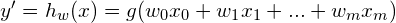or just: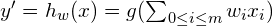where:

• y’ is the predicted response value.
• m is the number of predictor variables.
• x is the vector of predictor variable values for each data point.
• w is the vector of weights (or coefficients) of Logistic Regression.

Note that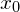is always set to be 1 for every data point. Thus,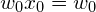and this value is called the intercept of the regressor.

The function g() is called Logistic Function (or another name, Sigmoid Function), which takes input as a continuous value (without bound) and outputs a value that is bounded in the range (0, 1).

Below are the form and the plot of g().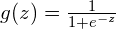Any data point that has output value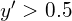is classified as 1-class (or sometimes we say, positive, or +), while data points with value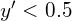are marked as 0-class (or negative, or -). An exception is when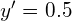, meaning the regressor doesn’t have any idea about how to classify this point at all. As this case is very rare (you can see that the probability of getting 0.5, an exact value, from a continuous space is 0), we take the convention that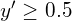indicates 1-class label.

Now we already get how a Logistic Regressor predicts a value, the difficulty becomes: how to train a Logistic Regressor (so that it has the best possible set of weights). To train means we make the model fit the data, in other words, we define an error term (or objective term) and try to minimize (or maximize) this term by adjusting the parameters of the model (for Logistic Regression, the parameters are the weights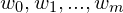). A very common way to define an objective term is by using Maximum Likelihood.

Notice that because the output value of the Logistic Function is in the range (0, 1), we can interpret this value as the probability of the data point to be in the Positive class. For example, if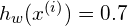, we say that the probability of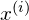belonging to the Positive class is 0.7, and thus the probability thatfalls into the Negative class is 0.3 . Writing this is the formula-form gives: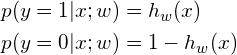or just: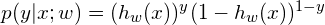(substituting y with 1 and 0 in the above formula will give you the equivalent of the higher above 2 formulas)

Assuming that the n training data points are independent, the Likelihood can be written as: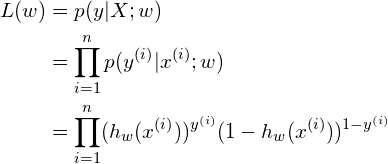We want to maximize this likelihood. However, being the multiplication of many values (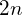values, with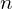is the number of data points) and each of these values is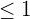, the resulting likelihood will likely be flawed/overflowed as number-representation in computers is limited. Another problem of Likelihood is that it is more complex for math-transformations when it comes to executing products.

To resolve these problems, instead of trying to maximize Likelihood, we can maximize the Log-Likelihood, which is a strictly increasing function of Likelihood (put differently, likelihood and log-likelihood increase or decrease together, thus maximizing log-likelihood is also equivalent to maximizing likelihood). The log-likelihood is: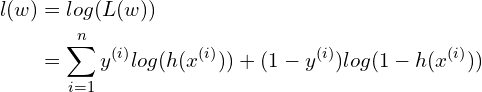As this is an optimization problem, many algorithms exist and are ready to be put in use (some are Simulated Annealing, Genetic Algorithm, Newton’s method). However, as we are working with Machine Learning, the most popular choice would be Gradient Descent (or Gradient Ascent).

Note that the Gradient Descent method is also sometimes called Gradient Ascent. They are basically the same with one trying to find the minimum (“descent” to the lowest position) and another finding the maximum (“ascent” to the highest position). These 2 can be simply converted to the other one with a flip (i.e. change the sign) in the objective function. In our current problem, as we try to maximize log-likelihood, we will use the Gradient Ascent method.

As using Gradient Descent/Ascent requires taking the derivatives, let us spend a little time examining the derivative of Logistic Function beforehand: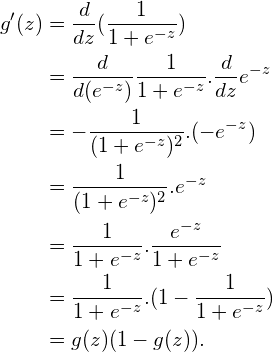This equation will be useful for our subsequent transformations.

Remember that the nature of Gradient Ascent is to iteratively move a little bit to the direction that seems to give a better result. To realize which direction and how much (small or steep) to move for a better result, we use the derivative at the current position. We also pre-determine a parameter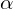(called the learning rate) to leverage how we adjust the parameters. Put differently, we update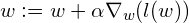.

Gradient Ascent (and also Gradient Descent) has some variants. Batch Gradient Ascent: we compute the value to update by using all the training points at once. Stochastic Gradient Descent: we choose one random data point at a time and execute the update for this data point only. Mini-batch Gradient Descent: each time we take a small subset of the data points to examine. Note that all these variants are iterative methods, i.e. we loop the process many times.

For simplicity, we will illustrate our work with the Stochastic Gradient Ascent (using 1 data point at a time). The other 2 are easily producible from this one. Also, we try to update 1 weight each, we call it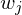(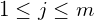).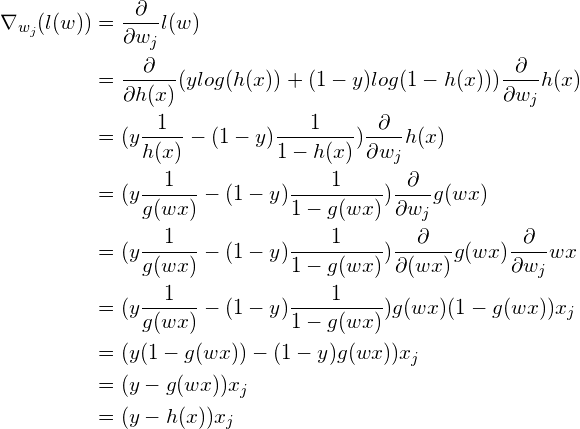Hence, we have, for each update: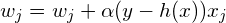Or more general: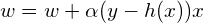The updating rule becomes very simple, the amount of update is just directly related to the difference between the real and predicted values. The rule is identical to of OLS even though the problem and algorithm are different. Actually, this is not a coincidence. A more detailed explaination about this phenomenon is given in another post about Generalized Linear Models.

Logistic Regression in Python

We can find an implementation of Logistic Regression in the sklearn library: sklearn.linear_model.LogisticRegression.

• Regularization is applied by default.
• The type of available regularization depends on the chosen solver.
• Multi-class classification is supported.
• Class weight is supported.
• We can get the coefficient weights and the number of iterations after the model finishes training.

Conclusion

I’m glad we are finally here!

In this blog, we talked about the theory behind Logistic Regression, the Log-likelihood maximization problem and how to solve this optimization stuff with the Gradient Accent method, along with how to run the algorithm automatically in Python.

There are more to come in the following blogs of this Logistic Regression series. Hence, if you enjoyed reading this blog, let’s go straight to the next one!

Goodbye and good luck!

References:

• Stoltzfus’s Logistic Regression: A brief Primer: link